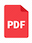## A Comparative Performance Analysis of ANN Algorithms for MPPT Energy Harvesting in Solar PV System

Authors: Rajib Baran Roy, Md. Rokonuzzaman, Nowshad Amin, Mahmuda Khatun Mishu, Sanath Alahakoon, Saifur Rahman, Nadarajah Mithulananthan, Kazi Sajedur Rahman, Mohammad Shakeri, Jagadeesh Pasupuleti
Publisher: IEEE Access, Vol. 9, 2021
Published on: 07/13/2021
DOI: https://doi-org.ezproxy.lib.vt.edu/10.1109/ACCESS.2021.3096864Download the Paper

Abstract:

In this paper, artificial neural network (ANN) based Levenberg-Marquardt (LM), Bayesian Regularization (BR) and Scaled Conjugate Gradient (SCG) algorithms are deployed in maximum power point tracking (MPPT) energy harvesting in solar photovoltaic (PV) system to forge a comparative performance analysis of the three different algorithms. A comparative analysis among the algorithms in terms of the performance of handling the trained dataset is presented. The MATLAB/Simulink environment is used to design the maximum power point tracking energy harvesting system and the artificial neural network toolbox is utilized to analyze the developed model. The proposed model is trained with 1000 dataset of solar irradiance, temperature, and voltages. Seventy percent data is used for training, while 15% data is employed for validation, and 15% data is utilized for testing. The trained datasets error histogram represents zero error in the training, validation, and test phase of data matching. The best validation performance is attained at 1000 epochs with nearly zero mean squared error where the trained data set is converged to the best training results. According to the results, the regression and gradient are 1, 1, 0.99 and 0.000078, 0.0000015739 and 0.26139 for Levenberg-Marquardt, Bayesian Regularization and Scaled Conjugate Gradient algorithms, respectively. The momentum parameters are 0.0000001 and 50000 for Levenberg-Marquardt and Bayesian Regularization algorithms, respectively, while the Scaled Conjugate Gradient algorithm does not have any momentum parameter. The Scaled Conjugate Gradient algorithm exhibit better performance compared to Levenberg-Marquardt and Bayesian Regularization algorithms. However, considering the dataset training, the correlation between input-output and error, the Levenberg-Marquardt algorithm performs better.

Keywords:
Solar photovoltaic (PV) , energy harvesting (EH) , maximum power point tracking (MPPT) , artificial neural network (ANN) , Levenberg-Marquardt (LM) , Bayesian regularization (BR) , scaled conjugate gradient (SCG)